## Introduction to Circles

The equation for a circle is an extension of the distance formula.

### Learning Objectives

Explain how the equation of a circle describes its properties

### Key Takeaways

#### Key Points

• A circle is defined as the set of points that are a fixed distance from a center point.
• The distance formula can be extended directly to the definition of a circle by noting that the radius is the distance between the center of a circle and the edge.
• The general equation for a circle, centered at $\left(a,b\right)$ with radius $r$, is the set of all points $\left(x,y\right)$ such that $\left(x−a\right)^2+\left(y−b\right)^2=r^2$.
• Pi ($\pi$) is the ratio of a circle’s circumference to its diameter.

#### Key Terms

• diameter: Two times the radius of a circle.
• area: The interior surface of a circle, given by $A = \pi r^2$.
• circle: A two-dimensional geometric figure, consisting of the set of all those points in a plane that are equally distant from another point.
• radius: A line segment between any point on the circumference of a circle and its center.

The definition of a circle is as simple as the shape. A circle is the set of all points that are at a certain distance from a center point. This definition is what gives us the concept of the radius of a circle, which is equal to that certain distance.

Since we know a circle is the set of points a fixed distance from a center point, let’s look at how we can construct a circle in a Cartesian coordinate plane with variables $x$ and $y$. To find a formula for this, suppose that the center is the point $\left(a,b\right)$. According to the distance formula, the distance $c$ from the point $\left(a,b\right)$ to any other point $\left(x,y\right)$ is:

$\displaystyle{c = \sqrt{ \left(x-a\right)^2 + \left(y-b\right)^2}}$

If we now square this equation on both sides, we have:

$\displaystyle{c^{2} = \left(x-a\right)^{2}+\left(y-b\right)^{2}}$

Remember that the distance between the center $\left(a,b\right)$ and any point $\left(x,y\right)$ on the circle is that fixed distance, which is called the radius. So let’s change this equation so that it uses $r$ instead of $c$.

$\displaystyle{r^{2} = \left(x-a\right)^{2}+\left(y-b\right)^{2}}$

This is the general formula for a circle with center $\left(a,b\right)$ and radius $r$. Notice that all we have done is slightly rearrange the distance formula equation.Graph of a circle: The circle with center $\left(a,b\right)$ is graphed in the Cartesian plane.

### Parts of a Circle

Now that we have an algebraic foundation for the circle, let’s connect it to what we already know about some different parts of the circle.

### Diameter

The diameter is any straight line that passes through the center of the circle. It is equal to twice the radius, so:

$d = 2r$

### Circumference

The circumference is the length of the path around the circle. Algebraically it is given by:

$c = 2\pi r$

or equivalently by $c = \pi d$. The number $\pi$ (pi) is defined by this relationship. It is the ratio of any circle’s circumference to its own diameter.

### Area

The area of a circle is given by:

$A = \pi r^2$

Notice that the radius is the only defining parameter for the size of any particular circle, and so it is the only variable that the area depends on.

## Introduction to Ellipses

Understand the equation of an ellipse as a stretched circle.

### Learning Objectives

Connect the equation for an ellipse to the equation for a circle with stretching factors

### Key Takeaways

#### Key Points

• An ellipse and a circle are both examples of conic sections.
• A circle is a special case of an ellipse, with the same radius for all points.
• By stretching a circle in the x or y direction, an ellipse is created.

An ellipse is one of the shapes called conic sections, which is formed by the intersection of a plane with a right circular cone. The general equation of an ellipse centered at $\left(h,k\right)$ is:

$\displaystyle{\frac{\left(x-h\right)^2}{a^2} + \frac{\left(y-k\right)^2}{b^2} = 1}$

when the major axis of the ellipse is horizontal.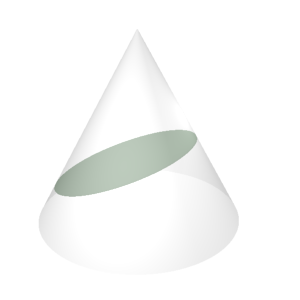Ellipse: An ellipse is a conic section, formed by the intersection of a plane with a right circular cone.

In most definitions of the conic sections, the circle is defined as a special case of the ellipse, when the plane is parallel to the base of the cone. However, it is also possible to begin with the definition of a circle and use graphical transformations to arrive at the general formula for an ellipse.

Recall that a circle is defined as the set of all points equidistant from a common center. For simplicity, we will choose that center to be $\left(0,0\right)$, the origin of the $x$–$y$ plane. Then we can write the equation of the circle in this way:

$x^2 + y^2 = r^2$

In this equation, $r$ is the radius of the circle. A circle has only one radius—the distance from the center to any point is the same. To change our circle into an ellipse, we will have to stretch or squeeze the circle so that the distances are no longer the same. First, let’s start with a specific circle that’s easy to work with, the circle centered at the origin with radius $1$.

$x^2 + y^2 = 1$

To make this into an ellipse, we must distort the circular shape so that it is no longer symmetric between $x$ and $y$. To do this, we introduce a scaling factor into one or both of the
$x$–$y$ coordinates. Let’s start by dividing all $x$ coordinates by a factor $a$, and therefore scaling the $x$ values. We simply substitute $\displaystyle{\frac{x}{a}}$ into the equation instead of $x$. Important note: We assume that $a > 1$.

$\displaystyle{\left(\frac{x}{a}\right)^2 + y^2 = 1}$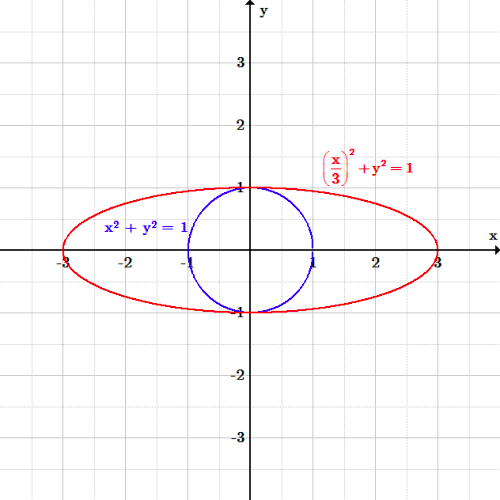Ellipse along $x$-axis: The ellipse $\left( \frac{x}{3} \right)^2 +y^2 = 1$ has been stretched along the $x$-axis by a factor of 3 as compared to the circle $x^2 + y^2 = 1$.

Every $x$-value that solved the old equation must now be multiplied by $a$ in order to solve the new equation. This has the effect of stretching the ellipse further out on the $x$-axis, because larger values of $x$ are now the solutions.

Similarly, we can scale all the values of $y$ by a factor $b$ (we also assume $b > 1$).

$\displaystyle {x^2 + \left(\frac{y}{b}\right)^2 = 1}$

Now all the $y$ values are stretched vertically, further away from the origin.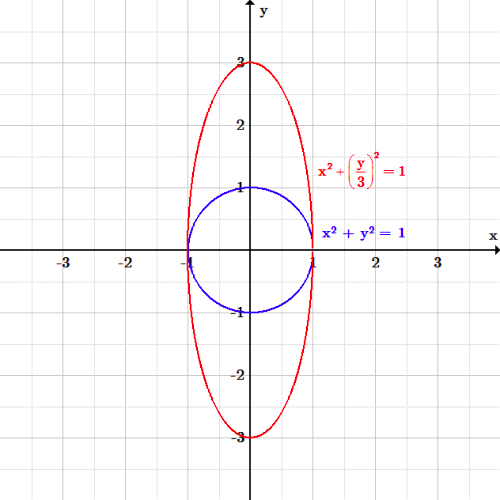Ellipse along $y$-axis: The ellipse $x^2 +\left( \frac{y}{3} \right)^2 = 1$ has been stretched along the $y$-axis by a factor of 3 as compared to the circle $x^2 + y^2 = 1$.

If we stretch in both the $x$ and $y$ directions and distribute the powers of two through the parentheses, we get:

$\displaystyle{\frac{x^2}{a^2} + \frac{y^2}{b^2} = 1}$

which is exactly the equation of a horizontal ellipse centered at the origin.

If we had used scaling factors that were less than one, it would have compressed the shape instead of stretching it further out.

## Parts of an Ellipse

Ellipses are one of the types of conic sections. The specific features of an ellipse can be determined from its equation.

### Learning Objectives

Discuss how the equation of an ellipse describes its properties

### Key Takeaways

#### Key Points

• An ellipse is formed by a plane intersecting a cone at an angle to its base.
• All ellipses have two focal points, or foci. The sum of the distances from every point on the ellipse to the two foci is a constant.
• All ellipses have a center and a major and minor axis.
• All ellipses have eccentricity values greater than or equal to zero, and less than one.

### Equation of an Ellipse

An ellipse is a conic section, formed by the intersection of a plane with a right circular cone. The standard form for the equation of the ellipse is:

$\displaystyle{\frac{\left(x-h\right)^2}{a^2} + \frac{\left(y-k\right)^2}{b^2} = 1}$

if the ellipse is oriented horizontally, and:

$\displaystyle{\frac{\left(y-k\right)^2}{a^2} + \frac{\left(x-h\right)^2}{b^2} = 1}$

if the ellipse is oriented vertically. We will use the horizontal case to demonstrate how to determine the properties of an ellipse from its equation, so that $a$ is associated with x-coordinates, and $b$ with y-coordinates. For a vertical ellipse, the association is reversed.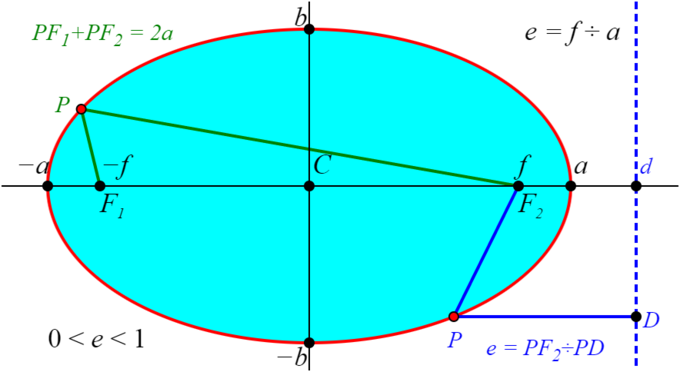Diagram of an ellipse: This diagram of a horizontal ellipse shows the ellipse itself in red, the center $C$ at the origin, the focal points at $\left(+f,0\right)$ and $\left(-f,0\right)$, the major axis vertices at $\left(+a,0\right)$ and $\left(-a,0\right)$, the minor axis vertices at $\left(0,+b\right)$ and $\left(0,-b\right)$. It also shows how the sum of the distances from any point on the ellipse to the two foci is a constant at $2a$ (the length of the major axis), and how the eccentricity is determined by the ratio of the distance from a point on the ellipse to one of the foci to the perpendicular distance from the point to a line $D$ called the directrix, outside the ellipse and perpendicular to the major axis.

### Center

The center of the ellipse has coordinates $(h,k)$.

### Major Axis

The major axis of the ellipse is the longest width across it. For a horizontal ellipse, that axis is parallel to the $x$-axis. The major axis has length $2a$. Its endpoints are the major axis vertices, with coordinates $(h \pm a, k)$.

### Minor Axis

The minor axis of the ellipse is the shortest width across it. For a horizontal ellipse, it is parallel to the $y$-axis. The minor axis has length $2b$. Its endpoints are the minor axis vertices, with coordinates $(h, k \pm b)$.

### Foci

The foci are two points inside the ellipse that characterize its shape and curvature. For a horizontal ellipse, the foci have coordinates $(h \pm c,k)$, where the focal length $c$ is given by

$c^2 = a^2 - b^2$

### Eccentricity

All conic sections have an eccentricity value, denoted $e$. All ellipses have eccentricities in the range $0 \leq e < 1$. An eccentricity of zero is the special case where the ellipse becomes a circle. An eccentricity of $1$ is a parabola, not an ellipse.

The eccentricity is defined as:

$\displaystyle{e = \frac{c}{a}}$

or, equivalently:

\displaystyle{ \begin{align} e &= \frac{\sqrt{a^2 - b^2}}{a} \\ &= \sqrt{ \frac{a^2 - b^2}{a^2} } \\ &= \sqrt{ 1 - \frac{b^2}{a^2}} \end{align} }

The orbits of the planets and their moons are ellipses with very low eccentricities, which is to say they are nearly circular. The orbits of comets around the sun can be much more eccentric. For comets and planets, the sun is located at one focus of their elliptical orbits.

## Applications of Circles and Ellipses

Circles and ellipses are encountered in everyday life, and knowing how to solve their equations is useful in many situations.

### Learning Objectives

Describe applications of the circle and ellipse formulas to real world problems

### Key Takeaways

#### Key Points

• The standard form of the equation for a circle is $\left(x-h\right)^2+\left(y-k\right)^2=r^2$, where $r$ is the radius, and $\left(h,k\right)$ is the center point coordinate.
• The standard form of the equation for an ellipse is $\displaystyle{\frac {\left(x-h\right)^2}{a^2}+\frac {\left(y-k\right)^2}{b^2}= 1}$, where $\left(h,k\right)$ is the center point coordinate, $2a$ is the length of the major/ minor axis, and $2b$ is the minor/major axis length.
• If $a>b$, the major axis is parallel to the $x$ axis. If $a<b$, the major axis is parallel to the $y$ axis (perpendicular to the $x$ axis).
• To determine the parameters of a circle or an ellipse, you must first put the equation into the standard form.

#### Key Terms

• ellipse: A closed curve, the locus of a point such that the sum of the distances from that point to two other fixed points (called the foci of the ellipse) is constant; equivalently, the conic section that is the intersection of a cone with a plane that does not intersect the base of the cone.
• circle: A two-dimensional geometric figure, consisting of the set of all those points in a plane that are equally distant from another point.

Circles are all around you in everyday life, from tires on cars to buttons on coats, as well as on the tops of bowls, glasses, and water bottles. Ellipses are less common. One example is the orbits of planets, but you should be able to find the area of a circle or an ellipse, or the circumference of a circle, based on information given to you in a problem. Circles and ellipses are examples of conic sections, which are curves formed by the intersection of a plane with a cone.

Some sample problems are shown below, with solutions worked out.

### Exercise 1

Let’s say you are a gardener, and you have just planted a lot of flowers that you want to water. The flower bed is 15 feet wide, and 15 feet long. You are using a circular sprinkler system, and the water reaches 6 feet out from the center. The sprinkler is located, from the bottom left corner of the bed, 7 feet up, and 6 feet over.

1. If the flower bed was a graph with the bottom left corner being the origin, what would the equation of the circle be?
2. What is the area being watered by the sprinkler?
3. What percentage of the garden that is being watered?

If the bottom left corner is the origin, it has coordinates $\left(0,0\right)$. From there the sprinkler is 6 feet over, so the $x$-coordinate of the center is 6. The sprinkler is 7 feet up, so the $y$-coordinate of the center is 7. Ergo, the center of the circle is at coordinate $\left(6,7\right)$. The water reaches 6 feet out from the sprinkler, so the circle radius is 6 feet. Therefore the equation of this circle is:

$\displaystyle{\left(x-6\right)^2+\left(y-7\right)^2=36}$

The area that is watered by the sprinkler can be labeled $A_{sprinkler}$, and is:

\displaystyle{ \begin{align} A_{sprinkler} &=\pi\cdot r^2\\ &=\pi\cdot 6^2\\ &=36\pi \end{align} }

The first step to finding the percentage of the garden that is being watered is to check that none of the water is falling outside the garden. The sprinkler is at coordinate $\left(6,7\right)$, and the radius of the sprinkler is 6 feet. You can test that the water does not fall outside the $15\times 15$ garden.

Once we know that the area that is watered is completely inside the garden, the percentage of the garden that is watered can be found by dividing the area watered by the total area of the garden, and then multiplying by $100\%$:

\displaystyle{ \begin{align} Percentage_{watered} &=\frac {A_{sprinkler}}{A_{flower bed}}\cdot 100\%\\ &=\frac {36\pi}{15^2}\cdot 100\%\\ &=\frac {113.1}{225}\cdot 100\%\\ &=50.3\% \end{align} }

### Exercise 2

Now, let’s take it the other way. $(x - 4)^2+(y+8)^2=49$ is the equation for a circle.

1. What are the coordinates of the center of the circle?
3. Draw the circle.
4. Find two points on the circle and plug them into the equation to make sure your drawing is correct.

The center of the circle can be found by comparing the equation in this exercise to the equation of a circle:

$\left(x-h\right)^2+\left(y-k\right)^2=r^2$

The center of the circle is at coordinate $\left(h,k\right)$, and so the center of the circle in this exercise is at $\left(4,-8\right)$.

The radius of the circle is $r$. From the equation of a circle, $\displaystyle{r^2=49}$. Therefore:

\displaystyle{ \begin{align} r^2 &= 49 \\ r &= \sqrt{49} \\ &= 7 \end{align} }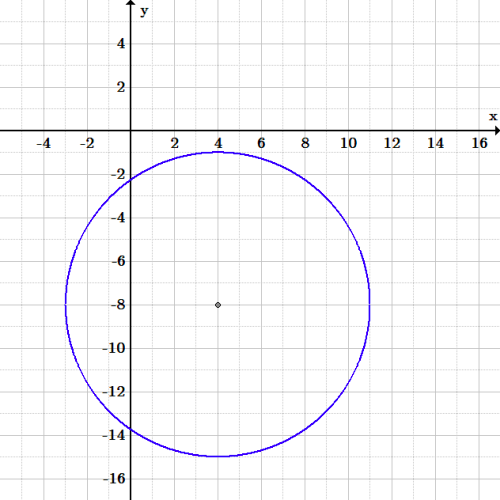Exercise 2 circle: Graph of the circle $(x – 4)^2+(y+8)^2=49$.

There are many points you could choose. For example, the point $\left(4, -1\right)$ is at the top of the circle. Plugging this into the equation, we get:

\displaystyle{ \begin{align} \left(x-h\right)^2+\left(y-k\right)^2 &=r^2 \\ (4-4)^2+(-1+8)^2 &= 49 \\ (0)^2 + (7)^2 &= 49 \\ 49 &= 49 \end{align} }

The left side is equal to the right side of the equation, and so this is a valid point on the circle.

The leftmost point on the circle is $(-3,-8)$. Plugging this in to the equation:

\displaystyle{ \begin{align} \left(x-h\right)^2+\left(y-k\right)^2 &=r^2 \\ \left(-3-4\right)^2+\left(-8+8\right)^2 &= 49 \\ (-7)^2 + (0)^2 &= 49 \\ 49 &= 49 \end{align} }

The left side is equal to the right side of the equation, and so this is a valid point on the circle.

### Exercise 3

1. Put $2x^2+2y^2+8x+24y+60=0$ into the standard circle form:         $(x-h)^2+(y-k)^2=r^2$.
2. What is the center and the radius of the circle?
3. Draw the circle.
4. Find two points on the circle and plug them into the equation to make sure your drawing is correct.

First, divide the equation by the coefficient of $x^2$ and $y^2$, which is $2$:

\displaystyle{ \begin{align} 2x^2+2y^2+8x+24y+60 &=0 \\ \frac{2x^2+2y^2+8x+24y+60}{2} &=\frac{0}{2} \\ \frac{2x^2}{2}+\frac{2y^2}{2}+\frac{8x}{2}+\frac{24y}{2}+\frac{60}{2} &=\frac{0}{2} \\ x^2+y^2+4x+12y+30 &=0 \end{align} }

Next, collect $x$ and $y$ terms together, and bring the number to the right side of the equation:

$(x^2+4x)+(y^2+12y)=-30$

Now, complete the square in both parentheses, subtracting or adding the necessary constant to both sides of the equation:

\displaystyle{ \begin{align} (x^2+4x+4)+(y^2+12y+36) &=-30+4+36 \\ (x^2+4x+4)+(y^2+12y+36) &= 10 \end{align} }

Notice that each term is a perfect square, which gives:

$(x+2)^2+(y+6)^2=10$

This is now in the standard form for the equation of a circle.

The center of the circle is at coordinate $\left(h,k\right)$, and so the center of the circle in this exercise is at $\left(-2,-6\right)$.

The radius of the circle is $r$. From the equation of a circle, $\displaystyle{r^2=10}$. Therefore:

\displaystyle{ \begin{align} r^2 &= 10 \\ r &= \sqrt{10} \end{align} }

It is fine to leave the value of $r$ in this form.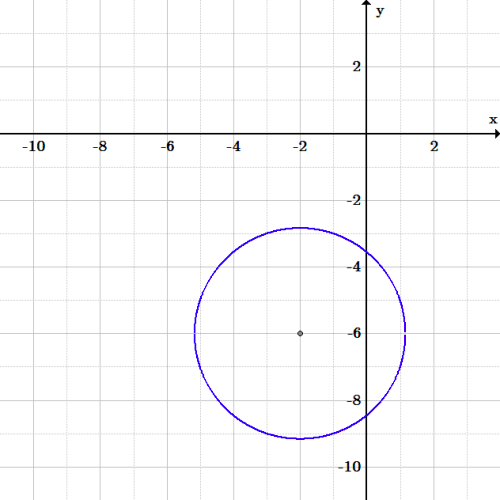Exercise 3 circle: Graph of the circle $2x^2+2y^2+8x+24y+60=0$.

There are many points you could choose. For example, $(-5,-5)$ and $(-3, -9)$:

\displaystyle{ \begin{align} (-5+2)^2 + (-5 + 6)^2 &= 10 \\ (-3)^2 + (1)^2 &= 10 \\ 9 + 1 &=10\\ 10 &= 10 \end{align} }

\displaystyle{ \begin{align} (-3+2)^2 + (-9+6)^2 &= 10\\ (-1)^2 + (-3)^2 &= 10 \\ 1 + 9 &= 10 \\ 10 &= 10 \end{align} }

Both are valid points on the circle.

### Exercise 4

1. Put the equation $\displaystyle{ \frac{4x^2}{9} + 25y^2 = 1 }$ into standard form.
2. What is the center?
3. How long is the major axis?
4. How long is the minor axis?
5. Graph it.

This almost looks like an ellipse in standard form, doesn’t it? It even has a number on the right side. But it isn’t. There is no room in the standard form for the values $4$ and $25$ in the numerators. How can we get rid of them to get into standard form?

Rewrite the left-hand term, $\displaystyle{\frac{4x^2}{9}}$, by dividing the top and bottom of the fraction by $4$. Leave the bottom as a fraction; don’t make it a decimal.

Rewrite the right-hand term, $25y^2$, as $\displaystyle{\frac{25y^2}{1}}$, and divide the top and bottom of the fraction by $25$. Leave the bottom as a fraction; don’t make it a decimal.

The equation is now:

\displaystyle{ \begin{align} \frac{4x^2}{9} + 25y^2 &= 1 \\ \frac{\frac{4x^2}{4}}{\frac{9}{4}} + \frac{\frac{25y^2}{25}}{\frac{1}{25}} &= 1 \\ \frac{x^2}{\frac{9}{4}} + \frac{y^2}{\frac{1}{25}} &= 1 \end{align} }

From the standard equation, $\displaystyle{\frac {\left(x-h\right)^2}{a^2}+\frac {\left(y-k\right)^2}{b^2}= 1}$, we know that the center is at $\left(h,k\right)$. Since these are both zero in this equation, the center is at $\left(0,0\right)$.

The major axis depends which is longer, $a$ or $b$. Let’s solve for both, and find out which is larger afterward.

\displaystyle{ \begin{align} a^2 &= \frac{9}{4} \\ a &= \frac{3}{2} \\ 2a &= \frac{6}{2} \\ &= 3 \end{align} }

\displaystyle{ \begin{align} b^2 &= \frac{1}{25} \\ b &= \frac{1}{5} \\ 2b &= \frac{2}{5} \end{align} }

Since $\displaystyle{3>\frac{2}{5}}$, $2a>2b$, and $2a$ is the major axis. The major axis length is $2a = 3$, and the minor axis length is $\displaystyle{2b = \frac{2}{5}}$.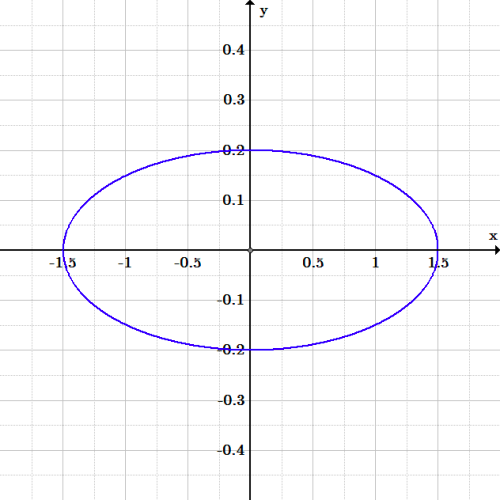Exercise 4 ellipse: Graph of the ellipse $\frac{4x^2}{9} + 25y^2 = 1$.